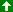Bubble Sort     Last updated on 2011/25551210, a full moon day;

The following sample code is based on Forte for Java, Community Edition v.1, 2000; Sun Microsystems;

class BubbleSortAlgorithm extends SortAlgorithm { // REMARK Sun's Java class
void sort(int a[]) throws Exception { // REMARK array a[ ] is defined as integer
for {int i = a.length, i>=0, i--) { // REMARK array a[]'s length is assigned as variable i; minus minus
boolean swapped = false; // REMARK not fuzzy set {... here, thus only true of false
for (int j = 0; j < i, j++) { // REMARK nested for { loop ... } therefore within the array a[]'s length; plus plus
if (stopRequested) { // REMARK since object has been throwable ...
return; // REMARK external class' stopRequested become true
}
if (a[j] > a[j + 1]) { // REMARK array a[]'s nested value
int T = a[j]; // REMARK also see: MSDN's <T>
a[j] = a[j + 1];
a[j + 1] = T;
swapped = true;
}
pause(i, j); // REMARK also see: MSDN's wait, however "pause" is not "wait"
}
if (!swapped)
return; // REMARK function sort() does not return, because the function has been defined as "void"
}
} // REMARK Bubble Sort Sample Code ends here

IFFcode (snippets) also see: keyword;

...Up